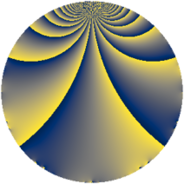# Properties

 Label 273.2.eLevel $273$ Weight $2$ Character orbit 273.e Rep. character $\chi_{273}(209,\cdot)$ Character field $\Q$ Dimension $32$ Newform subspaces $1$ Sturm bound $74$ Trace bound $0$

# Related objects

## Defining parameters

 Level: $$N$$ $$=$$ $$273 = 3 \cdot 7 \cdot 13$$ Weight: $$k$$ $$=$$ $$2$$ Character orbit: $$[\chi]$$ $$=$$ 273.e (of order $$2$$ and degree $$1$$) Character conductor: $$\operatorname{cond}(\chi)$$ $$=$$ $$21$$ Character field: $$\Q$$ Newform subspaces: $$1$$ Sturm bound: $$74$$ Trace bound: $$0$$

## Dimensions

The following table gives the dimensions of various subspaces of $$M_{2}(273, [\chi])$$.

Total New Old
Modular forms 40 32 8
Cusp forms 32 32 0
Eisenstein series 8 0 8

## Trace form

 $$32q - 32q^{4} + 4q^{7} - 8q^{9} + O(q^{10})$$ $$32q - 32q^{4} + 4q^{7} - 8q^{9} - 12q^{15} + 16q^{16} - 20q^{18} - 4q^{21} - 16q^{22} - 28q^{28} + 16q^{30} + 24q^{36} + 24q^{37} + 32q^{43} - 24q^{46} - 24q^{49} - 8q^{51} + 32q^{57} + 24q^{58} - 28q^{60} + 8q^{63} + 48q^{64} - 32q^{67} - 8q^{70} + 64q^{72} + 20q^{78} - 32q^{79} + 32q^{81} - 48q^{84} - 16q^{85} + 64q^{88} + 4q^{91} - 52q^{93} + 20q^{99} + O(q^{100})$$

## Decomposition of $$S_{2}^{\mathrm{new}}(273, [\chi])$$ into newform subspaces

Label Dim. $$A$$ Field CM Traces $q$-expansion
$$a_2$$ $$a_3$$ $$a_5$$ $$a_7$$
273.2.e.a $$32$$ $$2.180$$ None $$0$$ $$0$$ $$0$$ $$4$$